# Naive Bayes Introduction

## Introduction

I’m sure you guys have a mailing account, but do you know how it filters out spam emails? Well, it is done by a machine-learning algorithm known as the Naive Bayes algorithm.The Naive Bayes algorithm belongs to the family of Supervised Learning Algorithms. This algorithm is established from the Bayes theorem and used to solve classification problems using a probabilistic method. Some popular examples of the Naive Bayes algorithm are Spam filtration, text classification, a recommendation system, and sentimental analysis.

Let us understand how the classifier works with an example.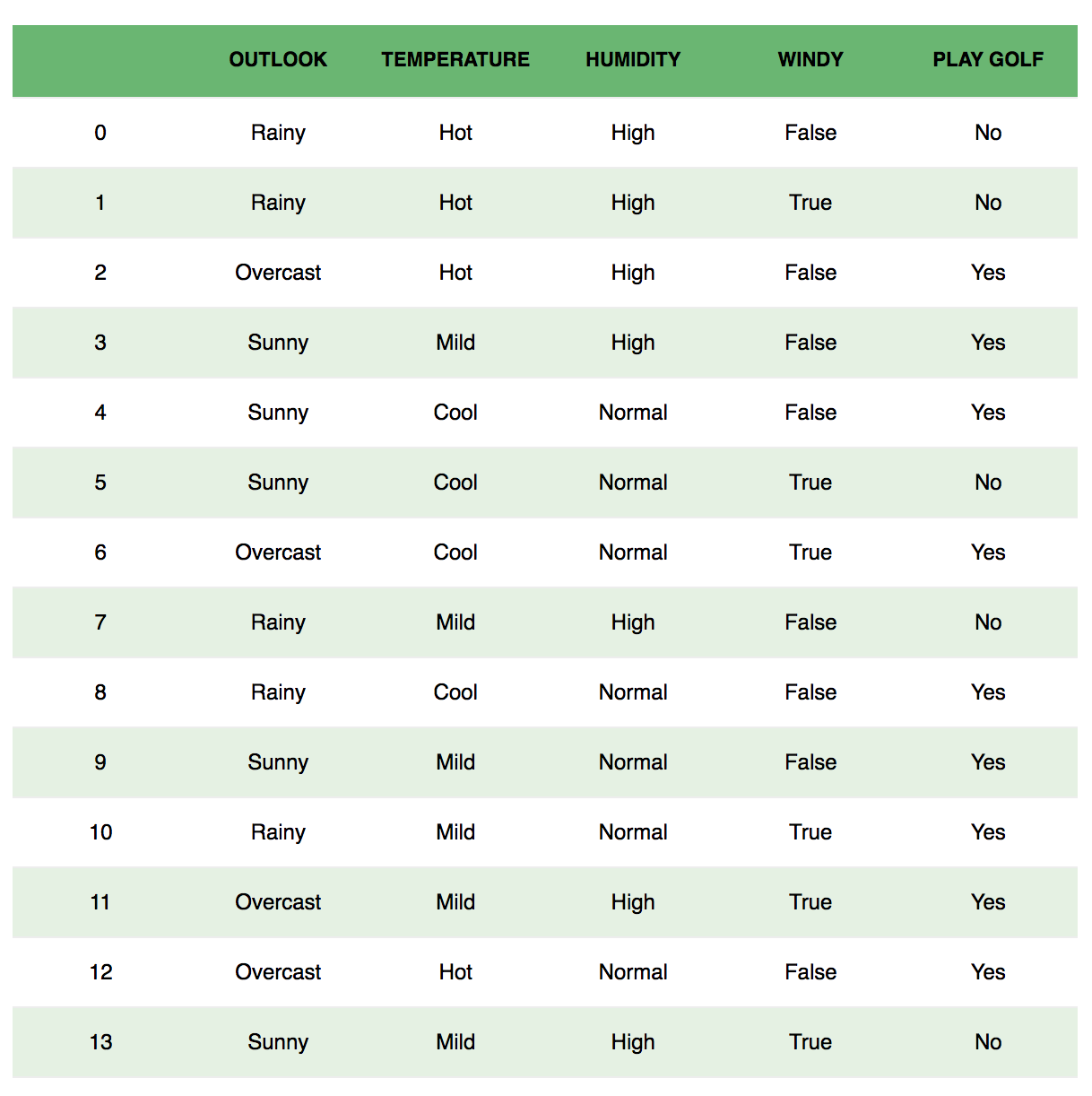The above dataset is fictional. We can see that the data is divided into two parts, namely, feature matrix and response vector:

• Feature matrices are the vectors/rows involving all the dependent features. In this dataset, weather, temperature, humidity, and wind are the feature matrix vectors.
• Response vectors are the class variables, i.e., the predictions or the output made for each row of the feature matrix. In this dataset, play golf is the response vector.

## Assumptions of Naive Bayes Classifier

After we have studied the dataset and understood the feature matrix and response vectors, it is vital to understand the assumptions made by the Naive Bayes classifier. The two main hypotheses are that the vectors are independent of each other, and there is no correlation. Another assumption is that all the features play an equal role, i.e., all the vectors are equally crucial for the prediction.

As in our dataset, the fact that outlook raining does not affect the temperature or windiness shows that our dataset is not correlated. Also, all the features selected are equally essential to predict whether to play golf or not.

Now, before jumping to the Naive Bayes Classifier algorithm, let us understand the Bayes Theorem.

## What is Bayes Theorem?

Bayes Theorem is a mathematical representation for determining conditional probability. It is the probability of an event occurring based on the previous occurring outcome. Bayes theorem is also called Bayes Rule or Bayes Law and is the foundation of Bayesian statistics. The mathematical formula for Bayes Theorem is: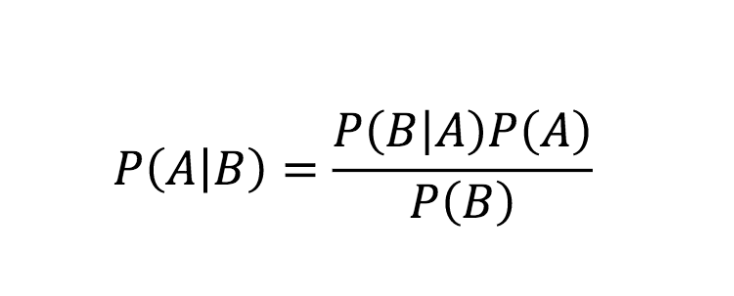P(A/B)- the probability of event A occurring, given event B has already occurred.

P(B/A)- the probability of event B occurring, given event A has already occurred.

P(A)- the probability of event A occurring

P(B)- the possibility of event B occurring

### What are we trying to calculate?

So basically, we are trying to find the probability of event A happening, given that event B is true. The probability of event B occurring is termed as evidence here. A's probability, i.e., P(A), is the probability/ priori of event A happening before the evidence is seen. Evidence is an attribute of an unknown instance. Here, P(A|B) is the probability of event A occurring after the evidence is seen, i.e., posteriori.

Therefore, the Bayes Theorem equation can be rewritten as: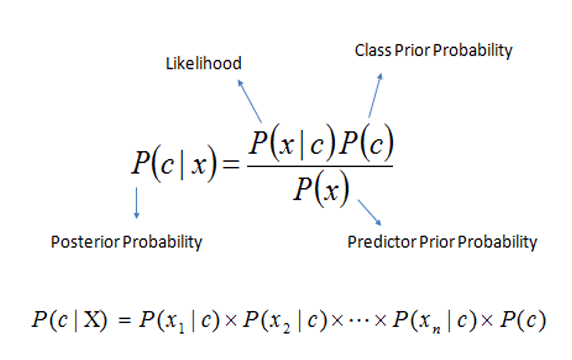Where,

P(c/x) is the posterior probability, i.e., the probability of event A occurring, given event B has already occurred.

P(x/c) is the likelihood probability, i.e., the probability of event B occurring, given event A has already occurred.

P(c) is the prior probability that is the probability of event A occurring

P(x) is the marginal probability that is the probability of event B occurring

Further, the above formula can be rewritten as: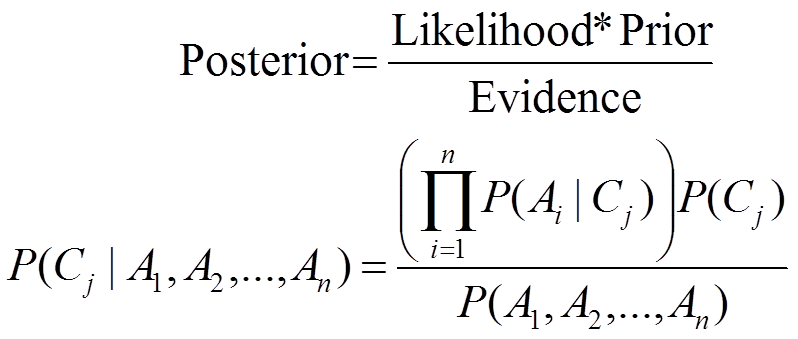### How does the Naive Bayes algorithm work?

Let us understand the functioning of the Naive Bayes algorithm using an example. Below is a training dataset of weather and corresponding target variable Play. Now we need to classify whether we should play or not based on the weather conditions.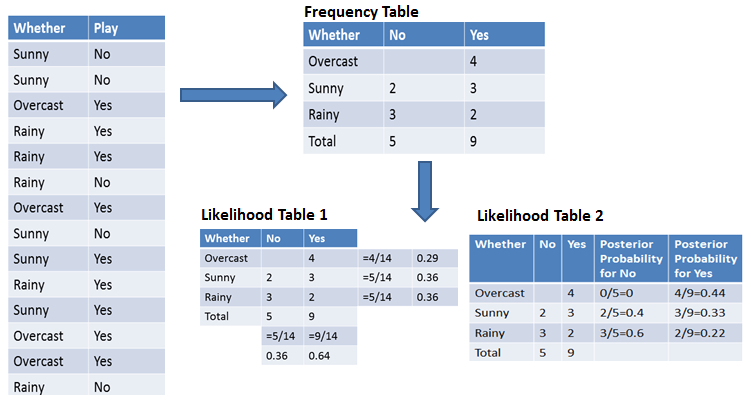The first step is to construct a frequency table of the given data. Next, we will create a likelihood table by finding the probabilities of sunny, rainy, etc. The final step is to use the Naive Bayes equation and find the probability of each category.

Problem statement: Players will play if the weather is sunny. Is this the correct statement?

We’ll solve the question by using the Naive Bayes formula.P(Yes / Sunny) = P( Sunny / Yes) * P(Yes) / P (Sunny)

Here we have,

P (Sunny /Yes) = 3/9 = 0.33,

P(Sunny) = 5/14 = 0.36,

P( Yes)= 9/14 = 0.64

Now, P (Yes | Sunny) = 0.33 * 0.64 / 0.36 = 0.60

which has a higher probability.

## Types of Naive Bayes Models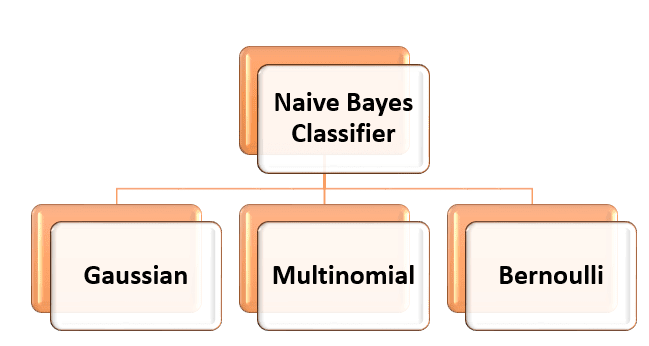There are three categories of Naive Bayes models.

### Gaussian Naive Bayes Model

This model assumes that normal distribution is followed by attributes. This means if the predictor takes a continuous value instead of a discrete one, the model assumes that these values are a sample of the Gaussian distribution; this distribution is also known as Normal distribution. When plotted, it gives a bell-shaped curve, which means that all the values are distributed across the mean value. The graph is represented like: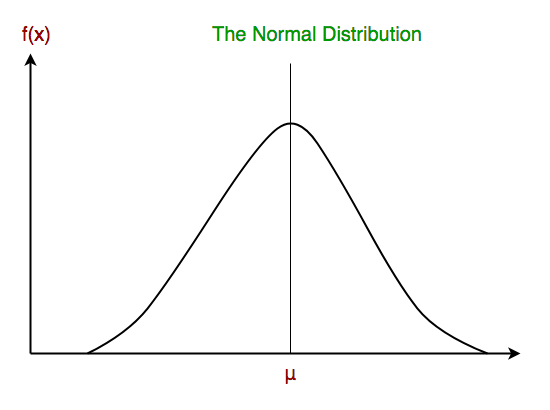This type of model is helpful for continuous values, and the assumption for this model justifies as constant values associated with each class are distributed normally. The following formula assumes the likelihood of the features: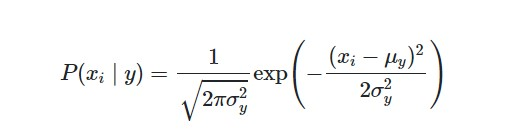Where,

y is the variance of y

Creating a simple Gaussian Naive Bayes assumes that a Gaussian distribution describes the data with no co-variance (independent) between dimensions. This model can be fit simply by finding the mean and standard deviation of the points within each label, all needed to define such a distribution.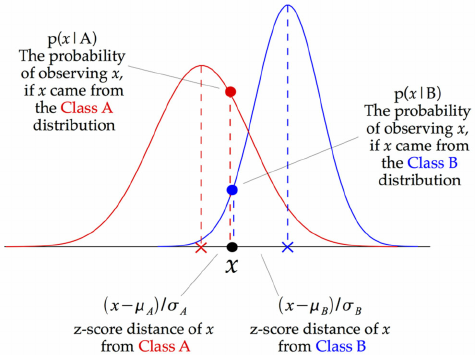This illustration indicates how a Gaussian Naive Bayes classifier works. At every data point, the z-score distance between that point and each class-mean is calculated, namely the distance from the class mean divided by the standard deviation of that class.

Let us have a look at a working example of Gaussian Naive Bayes code:

``````#importing the necessary libraries
import numpy as np
from sklearn.naive_bayes import GaussianNB
#Creating a sample dataset
X = np.array([[-1, -1], [-2, -1], [-3, -2], [1, 1], [2, 1], [3, 2]])
Y = np.array([1, 1, 1, 2, 2, 2])
print("Values in Array X:",X, "Values in Array Y:",Y, sep='\n')
#Creating the Gaussian Naive Bayes Classifier model with the inbuilt function.
model = GaussianNB()
model.fit(X, Y)
print("Predicted value for the input (-4,-7) is:",model.predict([[-4, -7]]))
print("Predicted value for the input (3,7) is:",model.predict([[3, 7]]))``````

This code will generate the following output:

``````Values in Array X:
[[-1 -1]
[-2 -1]
[-3 -2]
[ 1  1]
[ 2  1]
[ 3  2]]
Values in Array Y:
[1 1 1 2 2 2]
Predicted value for the input (-4,-7) is: 
Predicted value for the input (3,7) is: ``````

### Multinomial Naive Bayes Model

This type of classifier is used for document classification questions. It uses the frequency of words to anticipate. It predicts that a particular document would fit into which category, for example, Politics, novel, fiction, horror, etc.

It is helpful to model feature vectors where each value represents the number of occurrences of a term or its relative frequency. If the feature vectors have n elements and each of them can assume k different values with probability pk, then: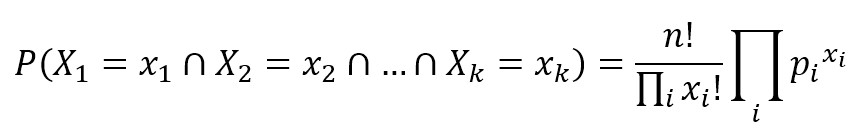Let us have a look at a working sample code:

``````#importing the necessary libraries
import numpy as np
from sklearn.naive_bayes import MultinomialNB
#Creating a sample dataset
rng = np.random.RandomState(1)
X = rng.randint(5, size=(6,6))
y = np.array([1, 2, 3, 4, 5, 6])
print("X:",X,"y:",y, sep='\n')
#Creating the Multinomial Naive Bayes Classifier model with the inbuilt function.
model = MultinomialNB()
model.fit(X, y)
print("Predicted value for the input value of (2,3) is:" ,model.predict(X[2:3]))``````

This code will generate the following output:

``````X:
[[3 4 0 1 3 0]
[0 1 4 4 1 2]
[4 2 4 3 4 2]
[4 2 4 1 1 0]
[1 1 1 1 0 4]
[1 0 0 3 2 1]]
y:
[1 2 3 4 5 6]
Predicted value for the input value of (2,3) is: ``````

### Bernoulli Naive Bayes Model

This type of classifier is used for discrete data, and it works on Bernoulli distribution. The primary feature of the Bernoulli Naive Bayes Classifier is that it accepts features only as binary values like, yes or no, success or failure, true or false, 0 or 1, and so on. Therefore, when the feature values are binary, we must use Bernoulli Naive Bayes classifier. The Bernoulli distribution can be represented in a mathematical formula as: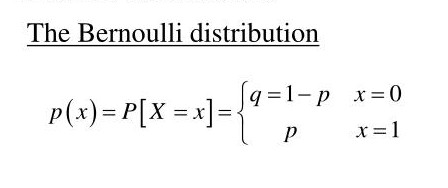Let us have a look at a working sample code:

``````#importing the necessary libraries
import numpy as np
from sklearn.naive_bayes import BernoulliNB
#Creating a sample dataset
rng = np.random.RandomState(1)
X = rng.randint(5, size=(6,6))
Y = np.array([1, 2, 3, 4, 5, 6])
print("X:",X,"y:",y, sep='\n')
#Creating the Bernoulli Naive Bayes Classifier model with the inbuilt function.
model = BernoulliNB()
model.fit(X, Y)
print("Predicted value for the input value of (5,6) is:",model.predict(X[5:6]))``````

The output of the code is as follows:

``````X:
[[3 4 0 1 3 0]
[0 1 4 4 1 2]
[4 2 4 3 4 2]
[4 2 4 1 1 0]
[1 1 1 1 0 4]
[1 0 0 3 2 1]]
y:
[1 2 3 4 5 6]
Predicted value for the input value of (5,6) is: ``````

### Advantages of Naive Bayes Classifier

• Naive Bayes is one of the most fast-moving and effortless Machine Learning algorithms to predict the class of a dataset.
• It can manage to perform Binary as well as Multi-class Classifications.
• It is best suited for multi-class predictions as compared to any other algorithm.
• The most popular practical implementation of this classifier is for text classification problems.

### Disadvantages of Naive Bayes Classifier

There are various disadvantages of Naive Bayesian classification,

• The data may not always be independent of each other.
• This algorithm can not be used for an imbalanced dataset.
• When we encounter a response vector in the test data for a particular class absent in the training data, we might end up with zero class probabilities; this is known as the Zero probability problem.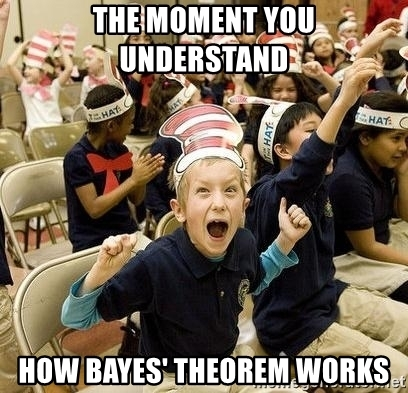## Applications of Naive Bayes Classifier

Naive Bayes Classifier has multiple real-life applications, to name a few are:

• Spam filtration
• Text classification
• Sentiment analysis
• Recommendation System
• Multi-class prediction

1. Does Naive Bayes work with missing values?
Ans: Missing values can be handled by the Naive Bayes algorithm. The features are handled separately at the time of model construction and prediction.

2. How do outliers affect naive Bayes?
Ans: The shape of Gaussian distribution will be affected by the outliers and would even affect the mean, standard deviation, and variance.

3. Can the Naive Bayes algorithm be used for regression?
Ans: Originally, Naive Bayes was built for classification problems only, but with some modifications, it can be used for regressions.

## Key takeaways

In this article, we learned about the theory of the Naive Bayes Algorithm, various types of Naive Bayes Algorithm, and the advantages and disadvantages. To build a career in Data Science? Check out our industry-oriented machine learning course curated by our faculty from Stanford University and Industry experts.